# Liouville Equation

Before we introduce MD techniques for the constant temperature and constant pressure ensembles, we have to derive the Liouville equation. It describes the evolution of the phase space distribution function for the conservative Hamiltonian system. In essence it is a continuity equation for the flux (evolution of ensemble in the phase space).

Consider phase space for the micro-canonical ensemble of the N-particle systems. Evolution of the ensemble is shown below: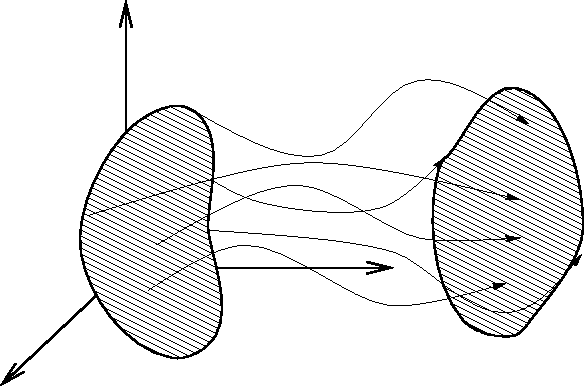As the number of systems in the ensemble is constant, we can write the continuity equation for density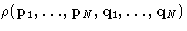in phase space: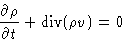(1)

For the flux in phase space, coordinates are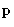's and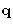's and velocities are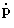's and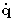's: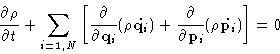(2)
Applying chain rule, we obtain:(3)
Using Hamilton equations of motion (remember, we consider a conservative system!):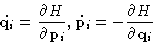(4)
we can see that: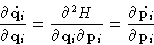(5)
Thus the second sum in equation (3) is zero, and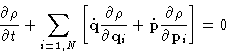(6)
This is Liouville equation.

The left hand side of is actually the full derivative of the distribution function, describing its change along the trajectories (flux lines). We proved Liouville theorem:

In conservative system the distribution function is constant along the trajectories, being an integral of motion.Next: Constant Temperature MD Up: Molecular Dynamics at Constant Previous: Molecular Dynamics at Constant

© 1997 Boris Veytsman and Michael Kotelyanskii
Tue Dec 2 20:24:47 EST 1997